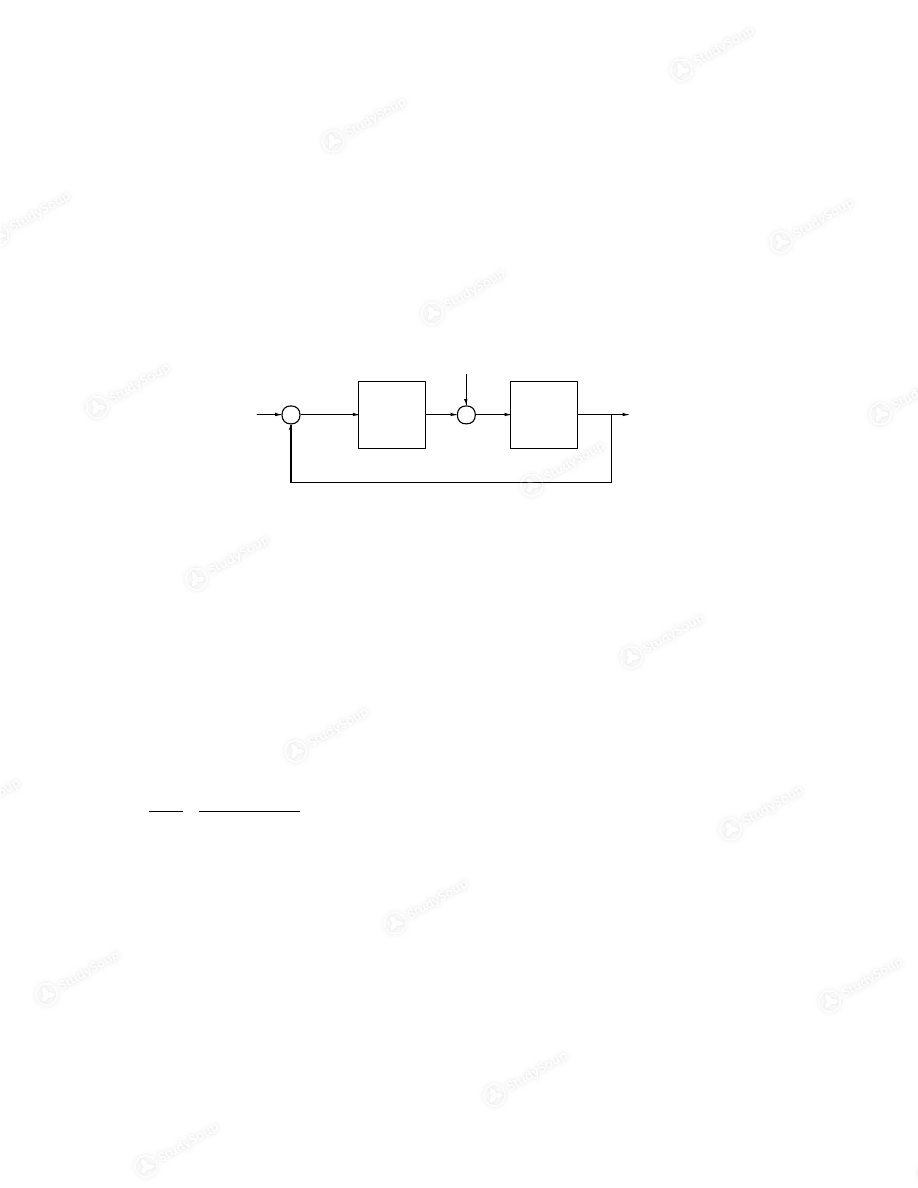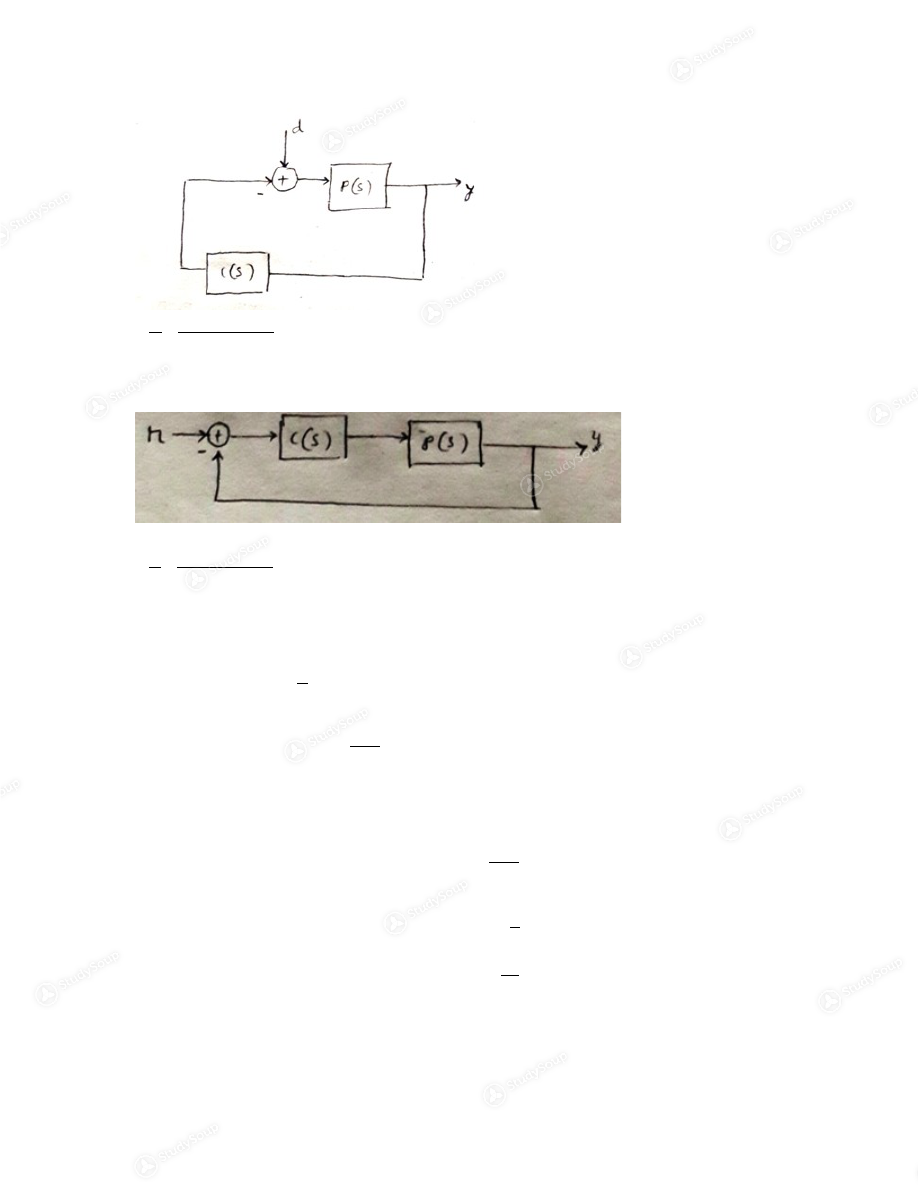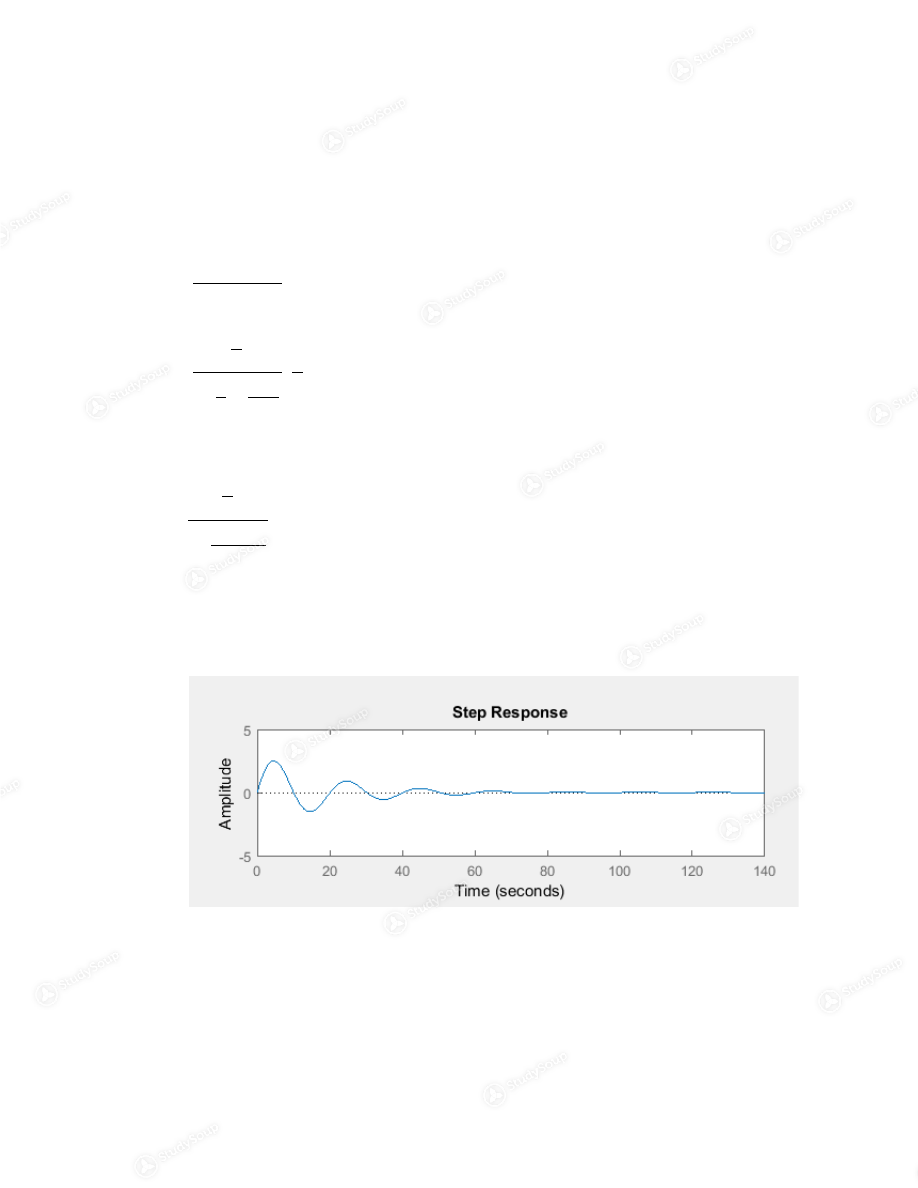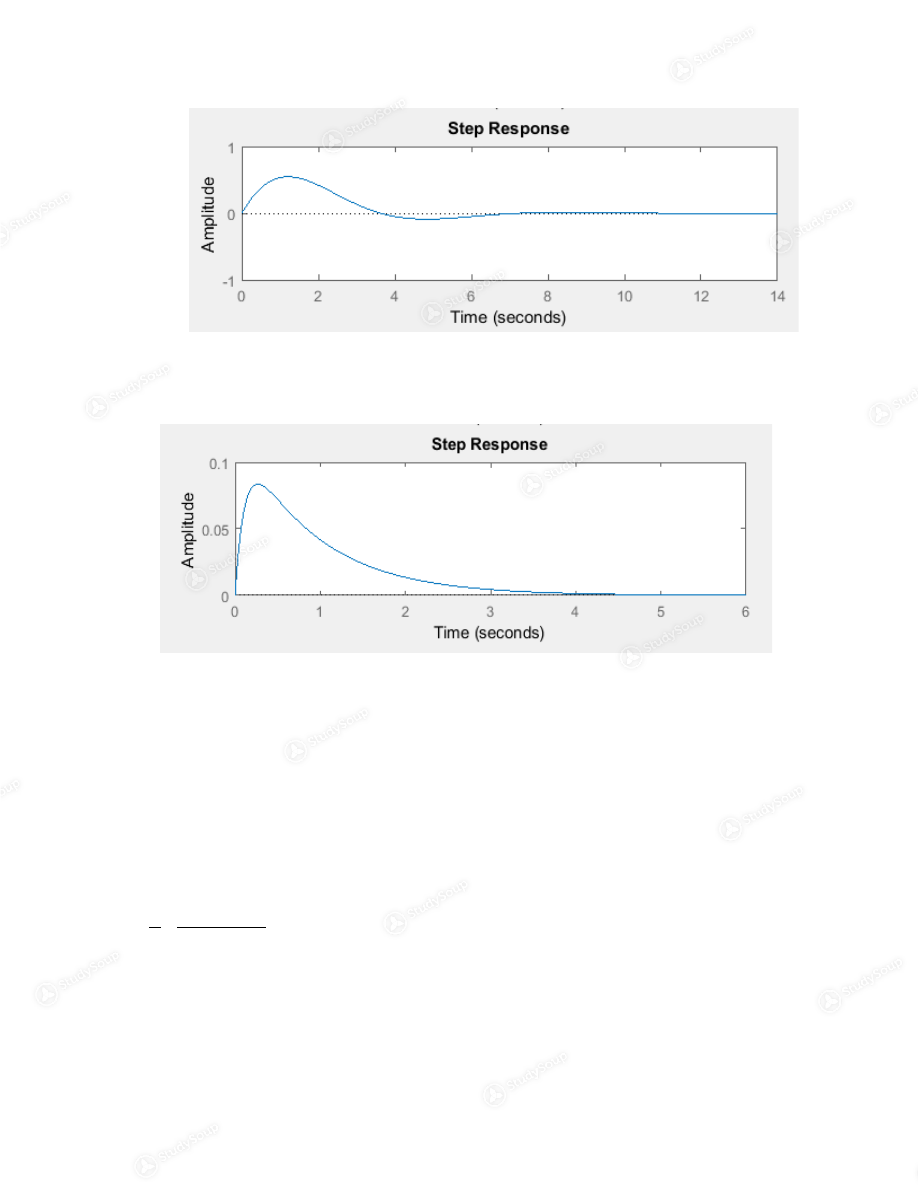Limited time offer 20% OFF StudySoup Subscription details

# CSU - Dominguez hills - MATLAB 2301 - Class Notes - Week 8

### Created by: Kahuhi Euticus Elite Notetaker

> > > > CSU - Dominguez hills - MATLAB 2301 - Class Notes - Week 8

CSU - Dominguez hills - MATLAB 2301 - Class Notes - Week 8

0 5 3 33 Reviews
This preview shows pages 1 - 5 of a 42 page document. to view the rest of the contentEEE 304 Lab Exercise 3: Linear Feedback In order to control the output of a system accurately and reliably, a control system is
required. Without a controller, the system may drift or become unstable. Take the
example of a car, where your foot is on the pedal and not making adjustments. If you
enter a downward slope, the car may speed up; that could be dangerous! Closing the
loop with a controller on the car, exactly what a cruise control does, will help keep
the speed steady. This is an example where closed-loop control can help get rid of
disturbances due to unmodeled dynamics.
In another example, consider a pendulum with one end attached to a motor. In order
to keep the pendulum upright many fast and accurate adjustments must be made. If
a   human   operator   attempts   to   do   this   and   the   pendulum   rod   is   too   short,   the
pendulum   will   fall   from   its   upright   position.   A   closed-loop   controller   with   fast
dynamics can however be able to stabilize the pendulum in the upright position. This
is an example where an “unstable” plant is stabilized via closed loop control. What
about   disturbances?   If   you   tap   the   pendulum   gently   it   may   even   reject   that
disturbance and manage to stay upright!
The above two examples serve as a brief introduction to the world of closed-loop
control and why it is necessary in automation. You should get familiar with the basic
concepts of linear feedback control systems from lectures, and this manual will not
focus on teaching, rather on assignments. Any MATLAB command you may not have
been introduced to  in the  previous  labs   will be  provided.  You  are  to  complete  all
exercises and produce their results as asked in your reports.EEE304 Lab 3 Answer Sheet Name:
Date:
Lab Description Write a paragraph explaining what you have learned from this lab exercise.  Task 1 For the control system shown in Fig. 1, + + ­ r e d y C(s) P(s) u Figure 1. A closed loop control system with disturbance where P(s) is the transfer function of the plant C(s) is the transfer function of the controller r is the reference signal e is the error signal u is the output signal from the controller d is the disturbance signal y is the output signal 1. Find the transfer function T dy  from the disturbance d to the output (assume r =  0). Find the transfer function T ry  from the reference r to the output (assume d =  0) . In general, the transfer function of a closed loop system is given by c ( s ) R ( s ) = G ( S ) ±G ( S ) H ( S ) Transfer function having d as input and y as output
Assuming
r=0 , theny d = P(s) 1+(s)(s) …….(i) Transfer function having r as input and y as output
Assuming
=0 , then G(s)=(sP(s), H (s)=1 y r = (s)(s) 1+(s)(s) 2. If the transfer functions of the plant and controller are given as Plant:  P ( s ) = 1 s , (e.g., a normalized description of car velocity with force as  the input; this can represent a cruise control system.) Controller:  C ( s ) = K s+z s , where K and z are constants. a) Verify that this is a PI controller. Show your work and state the expression for the  P and I gains in terms of K and z. Given controller as  C ( s ) = K s+z s C ( s ) = K ( 1+ z
s
) C ( s ) = + kz s Which is a proportional + an integral control
Proportional gain
¿ k Integral gain  ¿ kzb) Show that if the input d is a step disturbance and  C ( 0 ) = , the effect of d on y approaches zero as   t → ∞   and   (Hint:  Assume K,z > 0.  Use  the final value theorem.)   Demonstrate   your   result   by   choosing   the   following   different   sets   of
values for K and (varied by orders of magnitude) and plotting their closed-loop
step response. Include MATLAB code and plots. Discuss your observation. From equation (i) y ( s ) = P ( s ) 1+P ( s ) C ( s ) . d ( s ) When d(s) is step input y ( s ) = 1 s 1+ 1 s . k s+z s . 1 s y ( t →∞ ) = lim s=0 sy ( s ) ¿ lim s=0 1 s 1+ (s+z) s 2 = 0 1) K = 0.1, z = 0.1 A= tf([1 0] , [1 0.1 0.1]);    % Tdy with k=0.1 , z=0.1 subplot(311), step(A) 2) K = 1, z = 1 B= tf([1 0] , [1 1 1]);        % Tdy with k=1 , z=1 subplot(312), step(B)3) K = 10, z = 10 C= tf([1 0] , [1 10 10]);      % Tdy with k=10 , z=10 subplot(313), step(C) 4) c) Design a controller (select values of K and z) such that the following specifications are met: Target crossover frequency = 1 rad/s (approximately equal to the closed-loop
bandwidth).
Target phase margin is 60⁰.  1) Show all your work and demonstrate your design meets these specifications by the use of “ allmargin ” command on the open-loop transfer function L(s) = P(s)C(s). (hint: “help allmargin” in Matlab). Include MATLAB code and results. The transfer function is y r = C ( s ) P ( s ) 1+C ( s ) P ( s )

This is the end of the preview. Please to view the rest of the contentJoin more than 18,000+ college students at California State University - Dominguez Hills who use StudySoup to get ahead
##### Description: 1. Find the transfer function Tdy from the disturbance d to the output y (assume r = 0). Find the transfer function Try from the reference r to the output y (assume d = 0). If the transfer functions o
42 Pages 123 Views 98 Unlocks
• Notes, Study Guides, Flashcards + More!
Get Full Access to CSU - Dominguez hills - MAT 119 - Class Notes - Week 8
×
Get Full Access to CSU - Dominguez hills - MAT 119 - Class Notes - Week 8

I don't want to reset my password

Need help? Contact support

Need an Account? Is not associated with an account
We're here to help